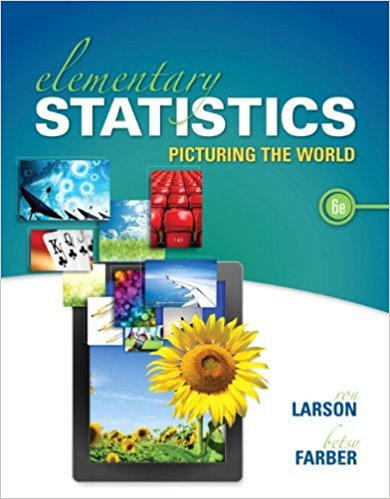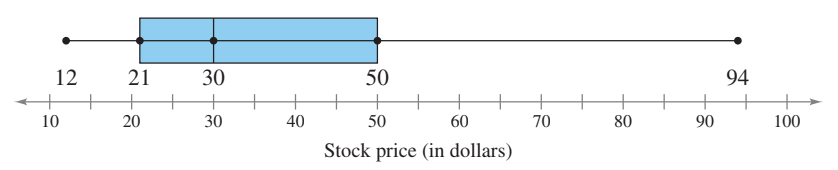×
Get Full Access to Elementary Statistics: Picturing The World - 6 Edition - Chapter 3.1 - Problem 76e
Get Full Access to Elementary Statistics: Picturing The World - 6 Edition - Chapter 3.1 - Problem 76e

×

Individual Stock Price An individual stock is selected atISBN: 9780321911216 66

Solution for problem 76E Chapter 3.1

Elementary Statistics: Picturing the World | 6th Edition

• Textbook Solutions
• 2901 Step-by-step solutions solved by professors and subject experts
• Get 24/7 help from StudySoup virtual teaching assistantsElementary Statistics: Picturing the World | 6th Edition

4 5 1 423 Reviews
15
1
Problem 76E

An individual stock is selected at random from the portfolio represented by the box-and-whisker plot shown. Find the probability that the stock price is (a) less than $21, (b) between$21 and $50, and (c)$30 or more.Step-by-Step Solution:

Solution :

Step 1 of 3:

Given the portfolio represented by the box-and-whisker plot is given below,Our goal is:

a). We need to find the probability that the stock price less than 21.

b). We need to find the probability that the stock price between 21 and 50.

c). We need to find the probability that the stock price 30 or more.

a). From the above plot, the following information is

The minimum value is 12 and thepercentile is 21.

The median (50th percentile) is 30

Then the maximum value is 94 and the 75th percentile is 50.

From the given information we know that to understand the data, this means 25% of the data is between the minimum and thepercentile.

Then 25% of the data is between thepercentile and median.

Now we have to find the probability less than 21.

So It's no coincidence that this is thepercentile.

Here 25% of the data is below 21 so the probability is 25%.

Therefore, the probability that the stock price less than 21 is 25 percent.

Step 2 of 3

Step 3 of 3

ISBN: 9780321911216

Unlock Textbook Solution